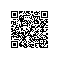# sql 分别按日,按周,按月,按季统计

--按日
select sum(consume),day([date]) from consume_record where year([date]) = '2006' group by day([date])

--按周quarter
select sum(consume),datename(week,[date]) from consume_record where year([date]) = '2006' group by datename(week,[date])

--按月
select sum(consume),month([date]) from consume_record where year([date]) = '2006' group by month([date])

--按季
select sum(consume),datename(quarter,[date]) from consume_record where year([date]) = '2006' group by datename(quarter,[date])使用钉钉扫一扫加入圈子
+ 订阅